# Trigonometric Functions - Online Test

Q1. In a triangle ABC, tan $,$ then tan  is equal to
Explaination / Solution:Q2. In a triangle ABC, if A =  and B =  then b + 2 c is equal to
Explaination / Solution: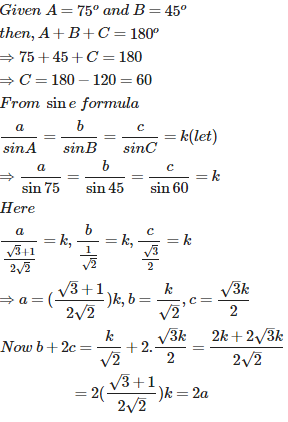Q3. If the sides of a triangle are 13, 7, 8 the greatest angle of the triangle is
Explaination / Solution:

Let the sides of the triangle be a=13,b=7 and c=8.

Since a  is the longest side the greatest angle is A.

Using Cosine rule, we have

Q4. There exists a triangle ABC satisfying the conditions
Explaination / Solution:

when k=1 we have

But we have  in a triangle the sum of the  three angles is 180 degrees ,so if B=90 degrees then $A<\frac{\pi }{2}$

Q5.

In a $,$ (b +c) cos A + (c + a) cos B + (a + b) cos C is equal to

Explaination / Solution: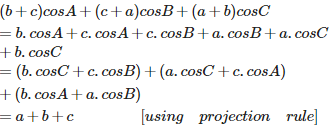Q6. The perimeter of a triangle ABC is 6 times the arithmetic mean of the sines of its angles. If the side b is 2, then the angle B is
Explaination / Solution:

Now when

Q7. In a triangle ABC,  is equal to
Explaination / Solution: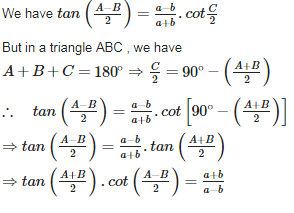Q8. The area of a triangle is  and its perimeter is 8 cm. The radius of its inscribed circle is
Explaination / Solution: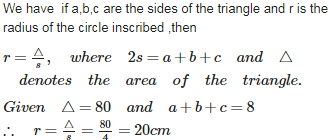Q9. If the angles of a triangle be in the ratio 1 : 4 : 5, then the ratio of the greatest side to the smallest side is
Explaination / Solution: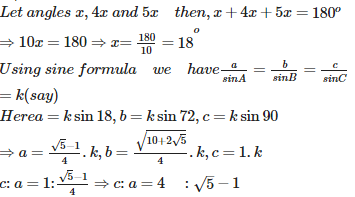Q10. If a = 4, b = 3 and A = then c is a root of the equation
Explaination / Solution:

Hence c is the root of the equation